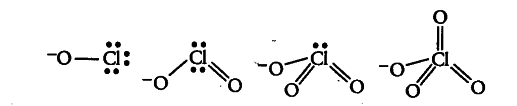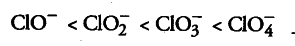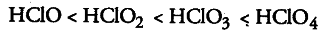# Explain why the stability of oxoacids of chlorine increases in the order given below?

Explain why the stability of oxoacids of chlorine increases in the order given below?
HCIO < \$HClO _{ 2 }\$ < \$HClO _{3}\$ < \$HClO _{4}\$

All these acids on losing a proton give their corresponding conjugate bases, i.e. {{ClO}^{-}}, Cl{ O }_{ 2 }^{ - } ,
Cl{ O }_{ 3 }^{ - } and
Cl{ O }_{ 4 }^{ - } Their structures are:Since, oxygen is more electronegative than chlorine, therefore dispersal of negative charge present on oxygen atom (singly bonded to Cl) increases as the number of oxygen atoms attached by a double bond to chlorine increases due to back bonding. Therefore, stability of ions will increase in the order given below:Due to increase in stability of conjugate base, acidic strength of corresponding acid increases in the same order as: